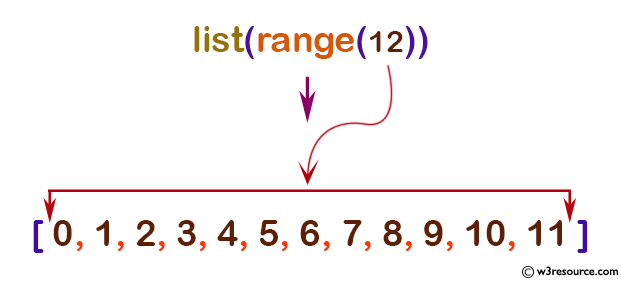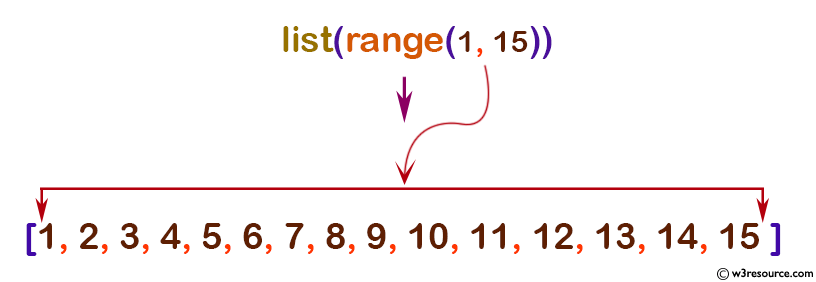# Python: range() function

## range() function

The range() function is used to get a sequence of numbers, starting from 0 by default, and increments by 1 by default, and ends at a specified number.

Note: Sequence Types - list, tuple, range etc.

Version:

(Python 3.2.5)

Syntax:

```range(stop)
range(start, stop[, step])
```

Parameter:

Name Description Required /
Optional
start

An integer number specify starting position. Default is 0.

Optional.
stop

An integer number specify end position. Default is 0.

Optional.
step Increment between each integer in the sequence. Default is 1 Optional.

Return value:

Returns an immutable sequence object of integers.

Example-1: Python range() function

``````# empty range
print(list(range(0)))

# using range(stop)
print(list(range(12)))

# using range(start, stop)
print(list(range(1, 15)))
``````

Output:

```[]
[0, 1, 2, 3, 4, 5, 6, 7, 8, 9, 10, 11]
[1, 2, 3, 4, 5, 6, 7, 8, 9, 10, 11, 12, 13, 14]
```

Pictorial Presentation:Pictorial Presentation:Example-2: Python range() function

``````print(range(10))

print(range(0, 30, 5))

print(range(0, -10, -1))

``````

Output:

```# range upto 10
print(range(10))

# range upto 30 but 5 step jumps
print(range(0, 30, 5))

# range() works with negative
print(range(0, -10, -1))
```

Example-3: Python range() works with negative step

``````# range upto 10
print(list(range(10)))

# range upto 30 but 5 step jumps
print(list(range(0, 30, 5)))

# range() works with negative step
print(list(range(0, -10, -1)))
``````

Output:

```[0, 1, 2, 3, 4, 5, 6, 7, 8, 9]
[0, 5, 10, 15, 20, 25]
[0, -1, -2, -3, -4, -5, -6, -7, -8, -9]
```

Python Code Editor:

Previous: property()
Next: repr()

Test your Python skills with w3resource's quiz

﻿

## Python: Tips of the Day

How do I check if a list is empty?

For example, if passed the following:

```a = []
if not a:
print("List is empty")
```

Ref: https://bit.ly/2A4JXx9